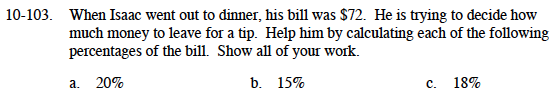### Home > MC1 > Chapter 10 > Lesson 10.2.3 > Problem10-103

10-103.10% of $72 is$7.20
20% = 2(10%)

10% of $72 is$7.20

10% of $72 is$7.20
1% of $72 is$0.72
18% = 2(10%) − 2(1%)

18% of $72 = 2($7.20) − 2($0.72) 18% of$72 = $14.40 −$1.44

18% of $72 is$12.96